# dsp.ArrayPlot

Display vectors or arrays

## Description

Display vectors or arrays where the data is uniformly spaced along the x-axis.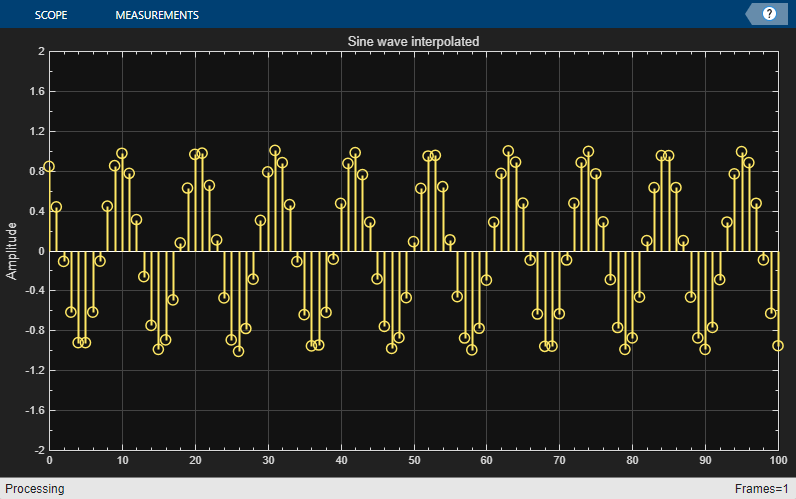## Creation

### Syntax

scope = dsp.ArrayPlot
scope = dsp.ArrayPlot(Name=Value)

### Description

example

scope = dsp.ArrayPlot creates an Array Plot object, scope.scope = dsp.ArrayPlot(Name=Value) sets properties using one or more name-value pairs. For example, scope = dsp.ArrayPlot(NumInputPorts=3)

## Properties

expand all

Most properties can be changed from the dsp.ArrayPlot UI.

### Plot Configuration

Number of input ports, specified as a positive integer. Each signal coming through a separate input becomes a separate channel in the scope. You must invoke the scope with the same number of inputs as the value of this property.

Specify whether to use the SampleIncrement and XOffset property values to determine spacing, or specify your own custom spacing. If you specify "Custom", you also must specify the CustomXData property values.

You can set this property only when creating the object.

#### Scope Window Use

Open the Plot tab, click Settings, and set X-Data Mode.

Data Types: char | string

Specify the desired x-data values as a row or column vector of length equal to the frame length of the individual inputs. If you use the default (empty vector) value, the x-data is uniformly spaced and set to (0:L–1), where L is the frame length.

You can set this property only when creating the object.

Example: scope = dsp.ArrayPlot(XDataMode="Custom",CustomXData=logspace(0,log10(44100/2),1024))

#### Scope Window Use

Open the Plot tab, click Settings, and set X-Data Mode to Custom and specify Custom X-Data.

#### Dependency

To use this property, set XDataMode to "Custom".

Specify the spacing between samples along the x-axis as a finite numeric scalar. The input signal is only y-axis data. x-axis data is set automatically based on the XOffset and SampleIncrement properties.

Example: When XOffset is 0 and SampleIncrement is 1, the x-axis values are set to 0, 1, 2, 3, 4, … .

Example: When XOffset is -1 and SampleIncrement is 0.25, the x-axis values are set to -1, -0.75, -0.5, -0.25, 0, … .

#### Scope Window Use

Open the Plot tab, click Settings, and set Sample Increment.

#### Dependency

To use this property, set XDataMode to 'Sample increment and X-offset'.

Display offset of x-axis, specified as a numeric scalar. x-axis data is set automatically based on both the SampleIncrement and XOffset values. The x-offset represents the first value on the x-axis.

Example: When XOffset is 0 and SampleIncrement is 1, the x-axis values are set to 0, 1, 2, 3, 4, … .

Example: When XOffset is -1 and SampleIncrement is 0.25, the x-axis values are set to -1, -0.75, -0.5, -0.25, 0, … .

#### Scope Window Use

Open the Plot tab, click Settings, and set X-Offset.

#### Dependency

To use this property, set XDataMode to "Sample increment and X-offset".

Specify whether the scale of the x-axis is "Linear" or "Log". If XOffset is a negative value, you cannot set this property to "Log".

#### Scope Window Use

Open the Plot tab, click Settings, and set XScale.

Data Types: char | string

Specify whether the scale of the y-axis is "Linear" or "Log".

#### Scope Window Use

Open the Plot tab, click Settings, and set YScale.

Data Types: char | string

Specify the type of plot to use for all the input signals displayed in the scope window:

• "Stem" – The scope displays the input signal as circles with vertical lines extending down to the x-axis at each of the sampled values.

• "Line" – The scope displays the input signal as lines connecting each of the sampled values.

• "Stairs" – The scope displays the input signal as a stair-step graph. A stair-step graph is made up of only horizontal lines and vertical lines. Each horizontal line represents the signal value for a discrete sample period and is connected to two vertical lines. Each vertical line represents a change in values occurring at a sample. Stair-step graphs are useful for drawing time history graphs of digitally sampled data.

#### Scope Window Use

Open the Settings and set Plot Type.

Specify when the scope scales the axes. Valid values are:

• "Auto" — The scope scales the axes as needed to fit the data, both during and after simulation.

• "Manual" — The scope does not scale the axes automatically.

• "OnceAtStop" — The scope scales the axes when the simulation stops.

• "Updates" — The scope scales the axes once after a set number of visual updates. The number of updates is determined by the value of the AxesScalingNumUpdates property.

You can set this property only when creating the object.

#### Scope Window Use

Hover over the array plot to see the zoom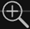, pan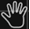, and autoscale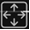buttons. You can also zoom and pan using your mouse.

Data Types: char | string

Specify the number of updates before scaling as a real, positive scalar integer.

#### Dependency

To enable this property, set AxesScaling to "Updates".

Data Types: double

### Measurements

Channel for which to obtain measurements, specified as a positive integer in the range [1 N], where N is the number of input channels.

#### Scope Window Use

Click the Measurements tab on the Array Plot toolstrip. In the Channel section, select a Channel.

Data Types: double

Cursor measurements to display screen or waveform cursors, specified as a CursorMeasurementsConfiguration object.

All CursorMeasurementsConfiguration properties are tunable.

#### Scope Window Use

Click the Measurements tab on the Array Plot toolstrip and modify the cursor measurements in the Cursors section.

Peak finder measurements to compute and display the largest calculated peak values, specified as a PeakFinderConfiguration object.

All PeakFinderConfiguration properties are tunable.

#### Scope Window Use

Click the Measurements tab on the Array Plot toolstrip and modify the peak finder measurements in the Peaks section.

Signal statistics measurements to compute and display signal statistics, specified as a SignalStatisticsConfiguration object.

All SignalStatisticsConfiguration properties are tunable.

#### Scope Window Use

Click the Measurements tab on the Array Plot toolstrip and modify the signal statistics measurements in the Statistics section.

### Visualization

Specify the name of the scope. This name appears as the title of the scope's figure window. To specify a title of a scope plot, use the Title property.

Data Types: char | string

Specify, in pixels, the size and location of the scope window as a four-element vector of the form [left bottom width height]. By default, the scope window appears in the center of your screen with a width of 800 pixels and height of 450 pixels. The default values for this property may change depending on your screen resolution.

Specify whether to display the scope in maximized-axes mode. In this mode, the axes are expanded to fit into the entire display. To conserve space, labels do not appear in each display. Instead, tick-mark values appear on top of the plotted data. You can select one of the following options:

• "Auto" — The axes appear maximized in all displays only if the Title and YLabel properties are empty for every display. If you enter any value in any display for either of these properties, the axes are not maximized.

• "On" — The axes appear maximized in all displays. Any values entered into the Title and YLabel properties are hidden.

• "Off" — None of the axes appear maximized.

#### Scope Window Use

Hover over the array plot to see the maximize axes button.

Data Types: char | string

Specify the display title as a character vector or string.

#### Scope Window Use

Open the Plot tab, click Settings, and set Title.

Data Types: char | string

To show a legend with the input names, set this property to true.

From the legend, you can control which signals are visible. This control is equivalent to changing the visibility in the Style dialog box. In the scope legend, click a signal name to hide the signal in the scope. To show the signal, click the signal name again. To show only one signal, right-click the signal name. To show all signals, press Esc.

Note

The legend only shows the first 20 signals. Any additional signals cannot be viewed or controlled from the legend.

#### Scope Window Use

On the Plot tab, click .

Data Types: logical

Specify the input channel names as a cell array of character vectors or an array of strings. The names appear in the legend, Settings, and Measurements panels. If you do not specify names, the channels are labeled as Channel 1, Channel 2, etc.

#### Dependency

To see channel names, set ShowLegend to true.

Data Types: char

Set this property to true to show grid lines on the plot.

#### Scope Window Use

Open the Plot tab, click Settings, and select Grid.

• true – The scope plots the magnitude and phase of the input signal on two separate axes within the same active display.

• false – The scope plots the real and imaginary parts of the input signal on two separate axes within the same active display.

This property is useful for complex-valued input signals. Turning on this property affects the phase for real-valued input signals. When the amplitude of the input signal is nonnegative, the phase is 0 degrees. When the amplitude of the input signal is negative, the phase is 180 degrees.

#### Scope Window Use

On the Plot tab, select the Magnitude Phase button.

Specify the text for the scope to display below the x-axis.

#### Scope Window Use

Open the Plot tab, click Settings, and set XLabel.

Data Types: char | string

Specify the text for the scope to display to the left of the y-axis.

#### Dependencies

This property applies only when PlotAsMagnitudePhase is false. When PlotAsMagnitudePhase is true, the two y-axis labels are read-only values "Magnitude" and "Phase", for the magnitude plot and the phase plot, respectively.

#### Scope Window Use

Open the Plot tab, click Settings, and set YLabel.

Data Types: char | string

Specify the y-axis limits as a two-element numeric vector, [ymin, ymax].

If PlotAsMagnitudePhase is false, the default is [-10,10]. If PlotAsMagnitudePhase is true, the default is [0,10].

#### Dependencies

When PlotAsMagnitudePhase is true, this property specifies the y-axis limits of only the magnitude plot. The y-axis limits of the phase plot are always [-180,180].

#### Scope Window Use

Open the Plot tab, click Settings, and set Y-Axis Limits as a two-element numeric vector.

## Usage

### Syntax

scope(signal)
scope(signal1,signal2,...,signalN)

### Description

example

scope(signal) displays the signal in the Array Plot.scope(signal1,signal2,...,signalN) displays multiple signals in the Array Plot. The signals can have a different number of channels and different frame lengths. 

### Input Arguments

expand all

Specify one or more input signals to visualize in the dsp.ArrayPlot. Signals can have a different number of channels and different frame lengths.

Example: scope(signal1,signal2)

#### UI Customization

To customize the style of signals on the array plot, open the and use the bottom row of options to select a signal and modify the style, width, color, and marker type.

Data Types: single | double | int8 | int16 | int32 | int64 | uint8 | uint16 | uint32 | uint64 | fi

## Object Functions

To use an object function, specify the object as the first input argument.

 generateScript Generate MATLAB script to create scope with current settings hide Hide scope window show Display scope window isVisible Determine visibility of scope step Run System object algorithm release Release resources and allow changes to System object property values and input characteristics reset Reset internal states of System object

If you want to restart the simulation from the beginning, call reset to clear the scope window displays. Do not call reset after calling release.

## Examples

collapse all

Create a new Array Plot object.

scope = dsp.ArrayPlot;

Configure the properties of the Array Plot object for a Gaussian distribution.

scope.YLimits = [0 1]; scope.XOffset = -2.5; scope.SampleIncrement = 0.1; scope.Title = "Gaussian distribution"; scope.XLabel = "X"; scope.YLabel = "f(X)";

Call the Array Plot object to plot a Gaussian distribution.

scope(exp(-(-2.5:.1:2.5).*(-2.5:.1:2.5))')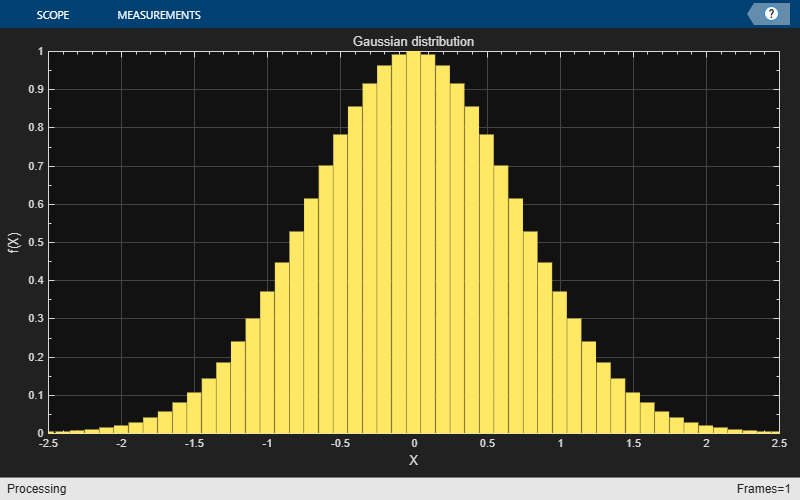You can scale the y-axis to show the plot on a log scale.

release(scope) scope.YScale = 'Log'; scope(exp(-(-2.5:.1:2.5).*(-2.5:.1:2.5))')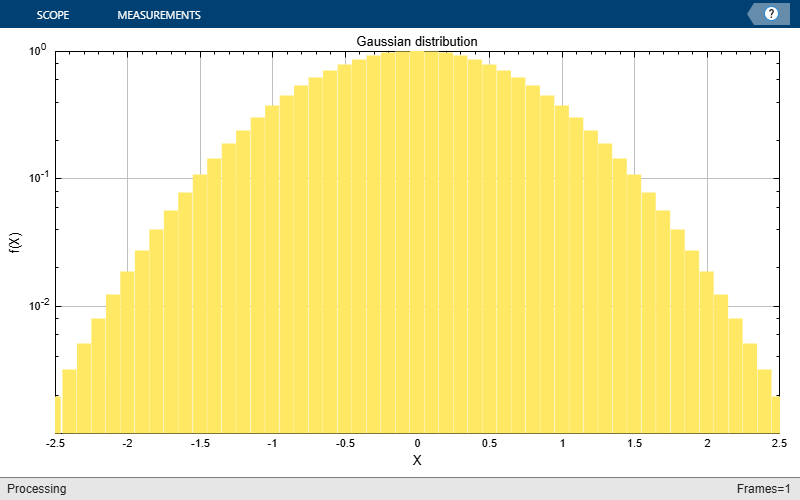View least mean squares (LMS) adaptive filter weights on the Array Plot figure. Watch the filter weights change as they adapt to filter a noisy input signal.

Create an LMS adaptive filter System object™.

lmsFilter = dsp.LMSFilter(40,Method="Normalized LMS",... StepSize=0.002); 

Create and configure a dsp.AudioFileReader System object to read the input signal from the specified audio file.

signalSource = dsp.AudioFileReader("dspafxf_8000.wav",... SamplesPerFrame=40, ... PlayCount=Inf,... OutputDataType="double"); 

Create and configure a dsp.FIRFilter System object to filter random white noise, creating colored noise.

firFilter = dsp.FIRFilter(Numerator=fir1(39,0.25)); 

Create and configure a dsp.ArrayPlot object to display the adaptive filter weights.

scope = dsp.ArrayPlot(XLabel="Filter Tap", ... YLabel="Filter Weight", ... YLimits=[-0.05 0.2]'); 

Plot the LMS filter weights as they adapt to a desired signal. Read from the audio file, produce random data, and filter the random data. Update the filter weights and plot the filter weights.

numplays = 0; while numplays < 3 [y, eof] = signalSource(); noise = rand(40,1); noisefilt = firFilter(noise); desired = y + noisefilt; [~, ~, wts] = lmsFilter(noise,desired); scope(wts); numplays = numplays + eof; end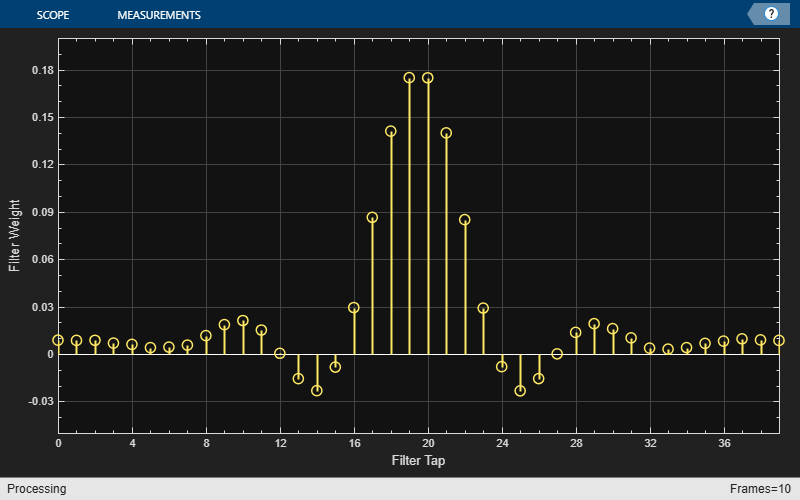Compute the power spectrum of a multichannel sinusoidal signal using the dsp.SpectrumEstimator System object™. You can get the vector of frequencies at which the spectrum is estimated using the getFrequencyVector function. To compute the resolution bandwidth of the estimate (RBW), use the getRBW function.

Generate a three-channel sinusoid sampled at 1 kHz. Specify sinusoidal frequencies of 100, 200, and 300 Hz. The second and third channels have their phases offset from the first by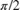and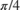, respectively.

sineSignal = dsp.SineWave('SamplesPerFrame',1000,'SampleRate',1000, ... 'Frequency',[100 200 300],'PhaseOffset',[0 pi/2 pi/4]); 

Estimate and plot the one-sided spectrum of the signal. Use the dsp.SpectrumEstimator object for the computation and the dsp.ArrayPlot for the plotting.

estimator = dsp.SpectrumEstimator('FrequencyRange','onesided'); plotter = dsp.ArrayPlot('PlotType','Line','YLimits',[0 0.75], ... 'YLabel','Power Spectrum (watts)','XLabel','Frequency (Hz)'); 

Step through to obtain the data streams and display the spectra of the three channels.

y = sineSignal(); pxx = estimator(y); plotter(pxx)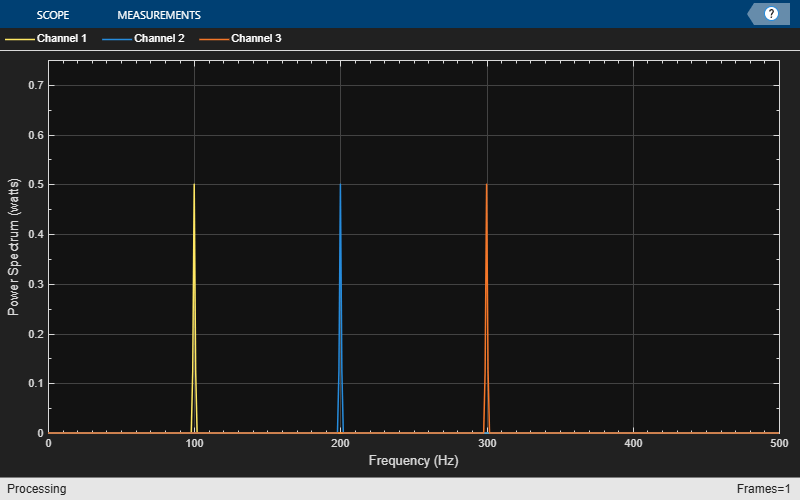Get the vector of frequencies at which the spectrum is estimated in Hz, using the getFrequencyVector function.

f = getFrequencyVector(estimator); 

Compute the resolution bandwidth (RBW) of the estimate using the getRBW function.

rbw = getRBW(estimator) 
rbw = 0.0015 

The resolution bandwidth of the signal power spectrum is 0.0015 Hz. This frequency is the smallest frequency that can be resolved on the spectrum.

Note: If you are using R2016a or an earlier release, replace each call to the object with the equivalent step syntax. For example, obj(x) becomes step(obj,x).

Generate a sine wave.

sineWave = dsp.SineWave('Frequency',100,... 'SampleRate',1000, ... 'SamplesPerFrame',1000); 

Use the spectrum estimator to compute the power spectrum and the max-hold spectrum of the sine wave. Use the Array Plot to display the spectra.

SE = dsp.SpectrumEstimator(... 'SampleRate',sineWave.SampleRate,... 'SpectrumType','Power','PowerUnits','dBm', ... 'FrequencyRange','centered',... 'OutputMaxHoldSpectrum',true); plotter = dsp.ArrayPlot('PlotType','Line',... 'XOffset',-500, ... 'YLimits',[-60 30],... 'Title','Power Spectrum of 100 Hz Sine Wave', ... 'YLabel','Power Spectrum (dBm)',... 'XLabel','Frequency (Hz)'); 

Add random noise to the sine wave. Stream in the data, and plot the power spectrum of the signal.

for ii = 1:10 x = sineWave() + 0.05*randn(1000,1); [Pxx,Pmax] = SE(x); plotter([Pxx Pmax]) end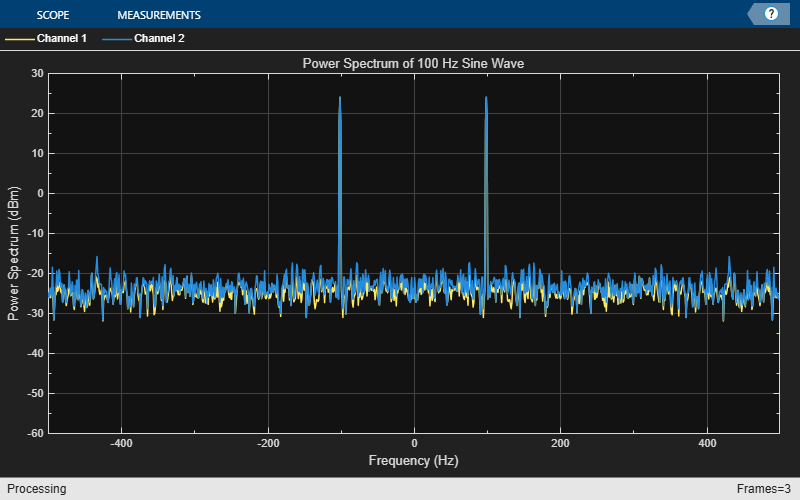## Version History

Introduced in R2013a

expand all